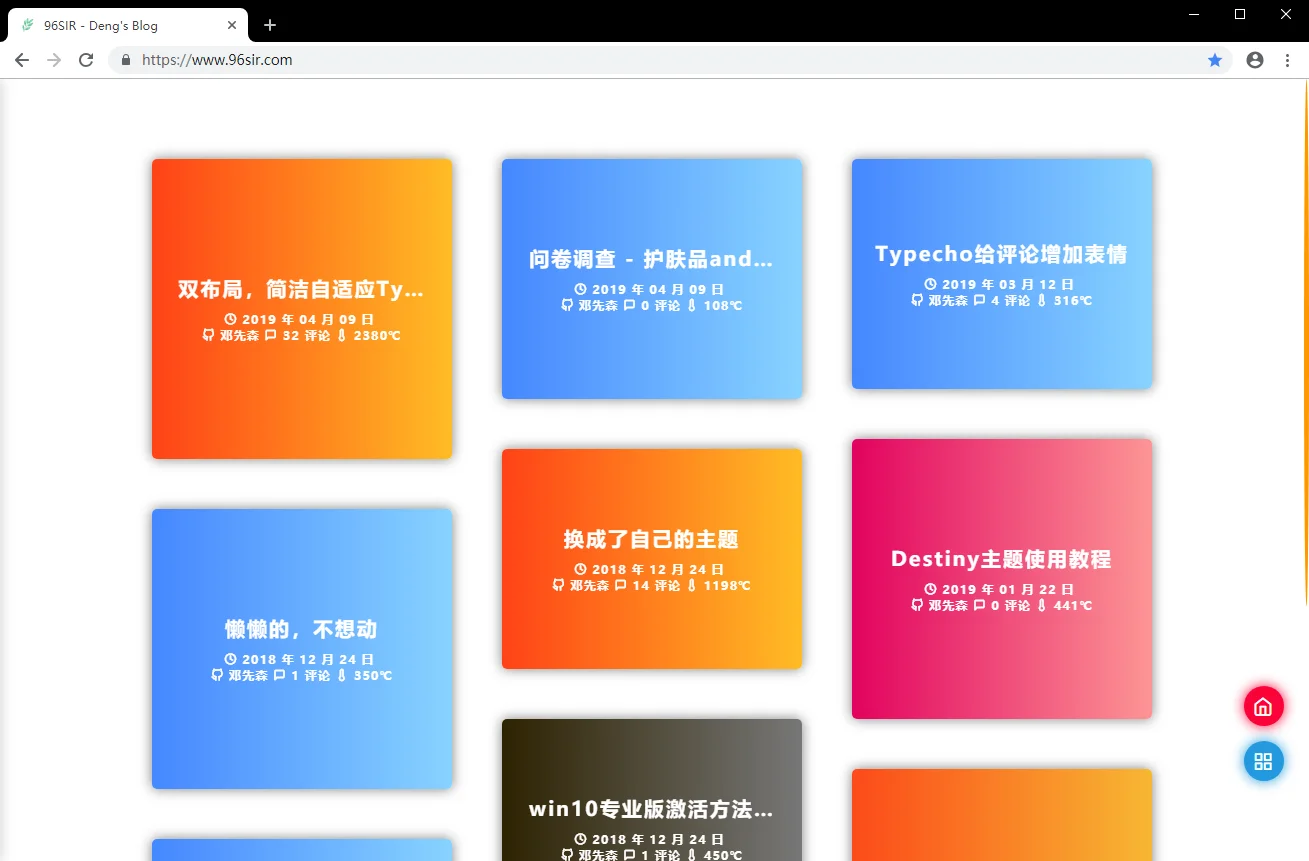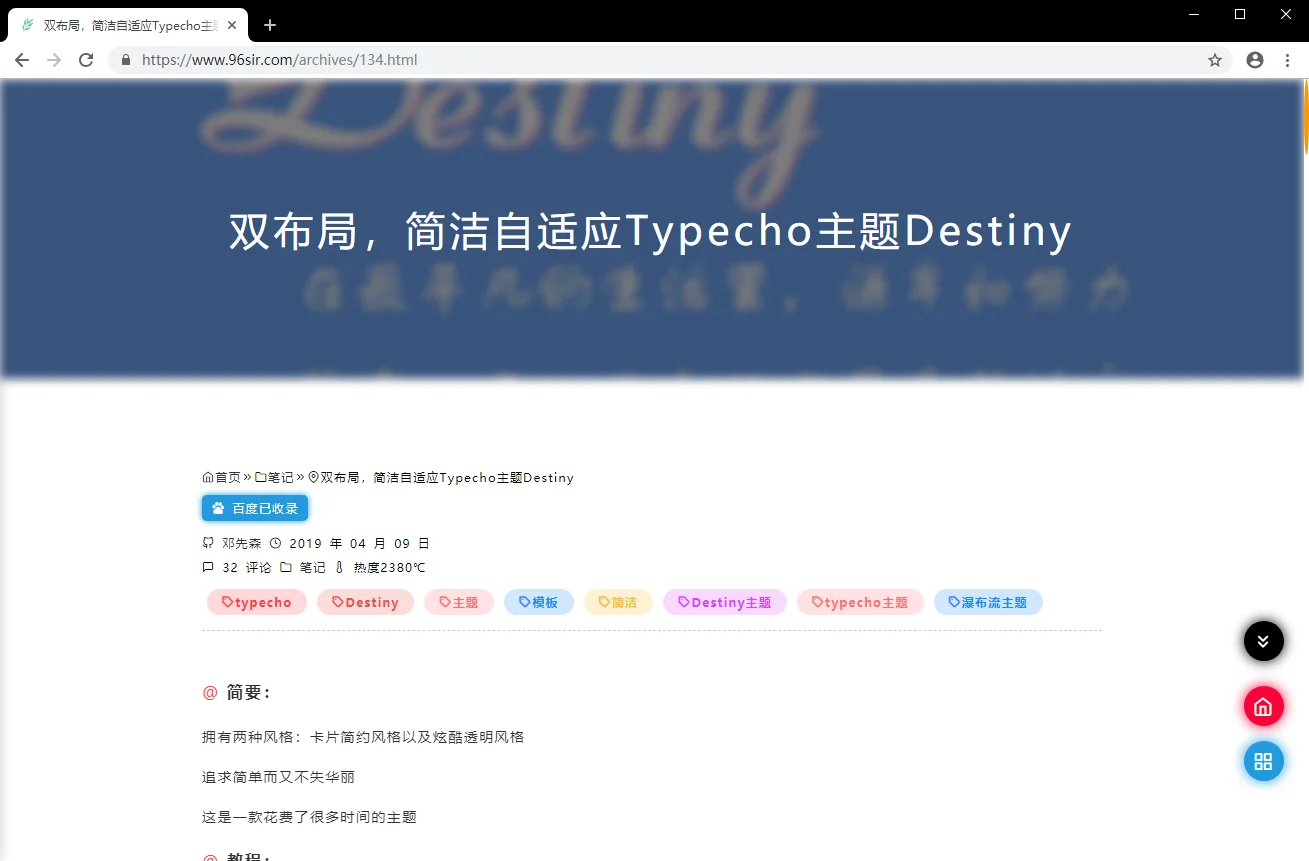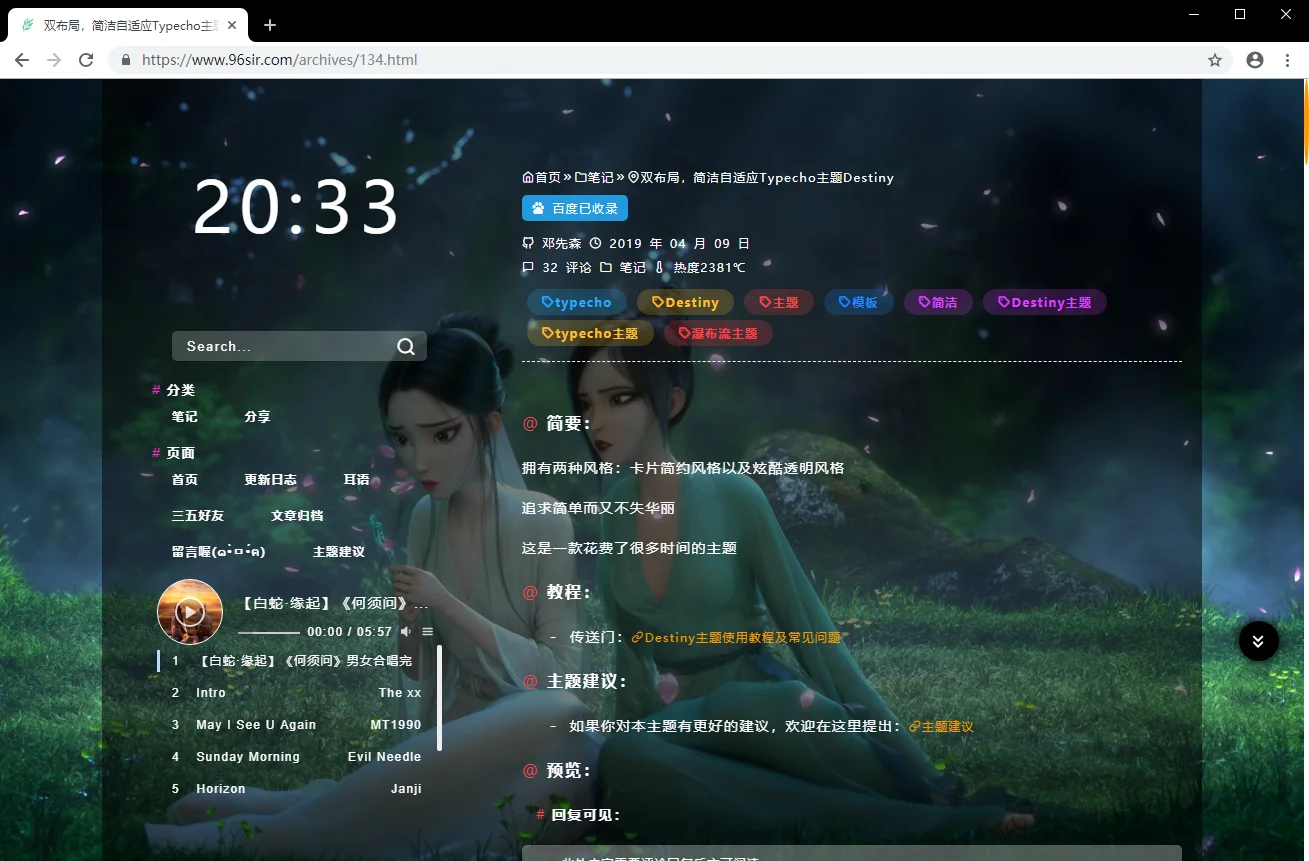## 主题展示

96Sir-Deng's Blog

## 预览

• 卡片简约风格：• 炫酷透明风格：• 简洁
• 响应式自适应
• 瀑布流排序布局
• 文章卡片
• 炫酷透明
• pjax刷新效果
• 文章图片灯箱
• 自带播放器

## 获取

• 主题售价48• 付款后发送付款截图以及联系方式（QQ）至邮箱[email protected]/* <![CDATA[ */!function(t,e,r,n,c,a,p){try{t=document.currentScript||function(){for(t=document.getElementsByTagName('script'),e=t.length;e--;)if(t[e].getAttribute('data-yjshash'))return t[e]}();if(t&&(c=t.previousSibling)){p=t.parentNode;if(a=c.getAttribute('data-yjsemail')){for(e='',r='0x'+a.substr(0,2)|0,n=2;a.length-n;n+=2)e+='%'+('0'+('0x'+a.substr(n,2)^r).toString(16)).slice(-2);p.replaceChild(document.createTextNode(decodeURIComponent(e)),c)}p.removeChild(t)}}catch(u){}}()/* ]]> */
• 作者将在24小时内回复（由于源码是可复制的，请购买的考虑清楚后再购买，恕不退款哈）
• 联系作者：传送门